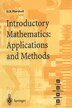Introductory Mathematics: Applications And Methods

byGordon S. Marshall, G. S. Marshall

Pricing and Purchase Info

$133.20 online$141.95 list price save 6%
Earn 666 plum® points

Prices and offers may vary in store

Quantity:

In stock online

Ships free on orders over \$25

Not available in stores

This book is aimed at undergraduate students embarking on the first year of a modular mathematics degree course. It is a self-contained textbook making it ideally suited to distance learning and a useful reference source for courses with the traditional lecture/tutorial structure. The theoretical content is firmly based but the principal focus is on techniques and applications. The important aims and objectives are presented clearly and then reinforced using complete worked solutions within the text. There is a natural increase in difficulty and understanding as each chapter progresses, always building upon the basic elements. It is assumed that the reader has studied elementary calculus at Advanced level and is at least familiar with the concept of function and has been exposed to basic differentiation and integration techniques. Although these are covered in the book they are presented as a refresher course to jog the student's memory rather than to introduce the topic for the first time. The early chapters cover the topics of matrix algebra, vector algebra and com­ plex numbers in sufficient depth for the student to feel comfortable -when they reappear later in the book. Subsequent chapters then build upon the student's 'A' level knowledge in the area of real variable calculus, including partial differentiation and mUltiple inte­ grals. The concluding chapter on differential equations motivates the student's learning by consideration of applications taken from both physical and eco­ nomic contexts.
Title:Introductory Mathematics: Applications And MethodsFormat:PaperbackDimensions:226 pagesPublished:April 28, 1998Publisher:Springer-Verlag/Sci-Tech/TradeLanguage:English

The following ISBNs are associated with this title:

ISBN - 10:3540761799

ISBN - 13:9783540761792

Reviews

1. Simultaneous Linear Equations.- 1.1 Introduction.- 1.2 The method of determinants.- 1.3 Gaussian elimination.- 1.4 Ill-conditioning.- 1.5 Matrices.- 1.5.1 Matrix notation.- 1.5.2 Matrix algebra.- 1.5.3 Inverse matrices.- 1.5.4 Matrix solution of linear equations.- 2. Vector Algebra.- 2.1 Introduction.- 2.2 Algebraic representation.- 2.3 Linear independence.- 2.4 The scalar product.- 2.5 The vector product.- 2.6 Triple products.- 2.7 Differentiation of vector functions.- 3. Complex Numbers.- 3.1 Introduction.- 3.2 Algebra of complex numbers.- 3.3 Graphical representation.- 3.4 Polar form.- 3.5 Exponential form.- 3.6 De Moivre's theorem.- 3.6.1 Roots of unity.- 3.6.2 Roots of complex numbers.- 4. Review of Differentiation Techniques.- 4.1 Introduction.- 4.2 Differentiation of standard functions,.- 4.3 Function of a function.- 4.4 The product rule.- 4.5 The quotient rule.- 4.6 Higher derivatives.- 4.7 Implicit differentiation.- 4.8 Logarithmic differentiation.- 5. Review of Integration Techniques.- 5.1 Introduction.- 5.2 Integration of standard functions.- 5.3 Integration by substitution.- 5.4 Integration using partial fractions.- 5.4.1 Introduction.- 5.4.2 Resolving into partial fractions.- 5.4.3 Repeated factors.- 5.5 Integration by parts.- 6. Applications of Differentiation.- 6.1 Introduction.- 6.2 Functions:.- 6.2.1 Increasing and decreasing functions.- 6.2.2 Relative maxima and minima.- 6.2.3 Concavity:.- 6.2.4 Points of inflection.- 6.2.5 Absolute maxima and minima.- 6.3 Curve sketching.- 6.4 Optimisation.- 6.5 Taylor series.- 7. Partial Differentiation.- 7.1 Introduction.- 7.2 Notation.- 7.3 Total differentials.- 7.4 The total derivative.- 7.5 Taylor series for functions of two variables.- 7.6 Maxima, minima and saddlepoints.- 7.6.1 Classification of critical points.- 7.7 Problems with constraints:.- 7.8 Lagrange multipliers.- 8. Multiple Integrals.- 8.1 Double integrais.- 8.1.1 Change of variable in double integrals.- 8.2 Triple integrais.- 9. Differential Equations.- 9.1 Introduction.- 9.2 First order differential equations.- 9.2.1 Equations of the form $$\frac{{dy}}{{dx}} = f\left( x \right)$$.- 9.2.2 Equations of the form mr$$\frac{{dy}}{{dx}} = ky$$.- 9.2.3 Variables separable.- 9.2.4 Integrating factors.- 9.3 Second order equations.- 9.3.1 Linear equations with constant coefficients.- 9.3.2 Solution of the homogenous equation.- 9.3.3 Simple harmonic motion.- 9.3.4 Damped SHM.- 9.3.5 Particular integrals involving elementary functions.- Solutions to Exercises.